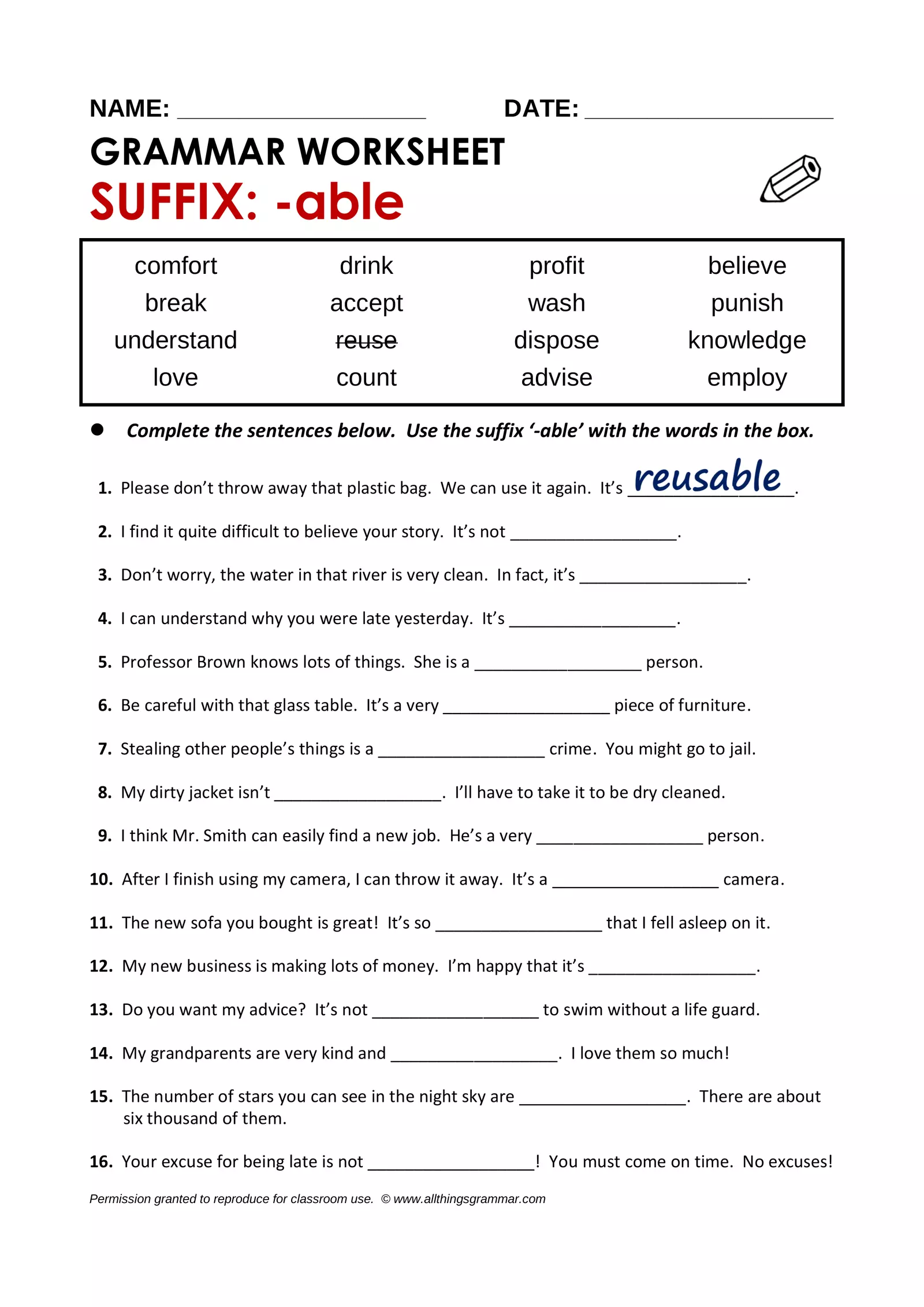# Atg worksheet suffix able

Created

GRAMMAR WORKSHEET ALL Things Grammar Grammar Focus Suffix: -able Level Intermediate to Advanced ANSWER KEY 1. reusable 9. employable 2. believable 10. disposable 3. drinkable 11. comfortable 4. understandable 12. profitable 5. knowledgeable 13. advisable 6. breakable 14. lovable 7. punishable 15. countable 8. washable 16. acceptable Grades as percentages 16 / 16 = 100% 15 / 16 = 94 14 / 16 = 86 13 / 16 = 81 12 / 16 = 75 11 / 16 = 69 10 / 16 = 63 9 / 16 = 56 8 / 16 = 50 7 / 16 = 44 6 / 16 = 38 5 / 16 = 31 4 / 16 = 25 3 / 16 = 19 2 / 16 = 13 1 / 16 = 6 0 / 16 = 0% Permission granted to reproduce for classroom use. © www.allthingsgrammar.com